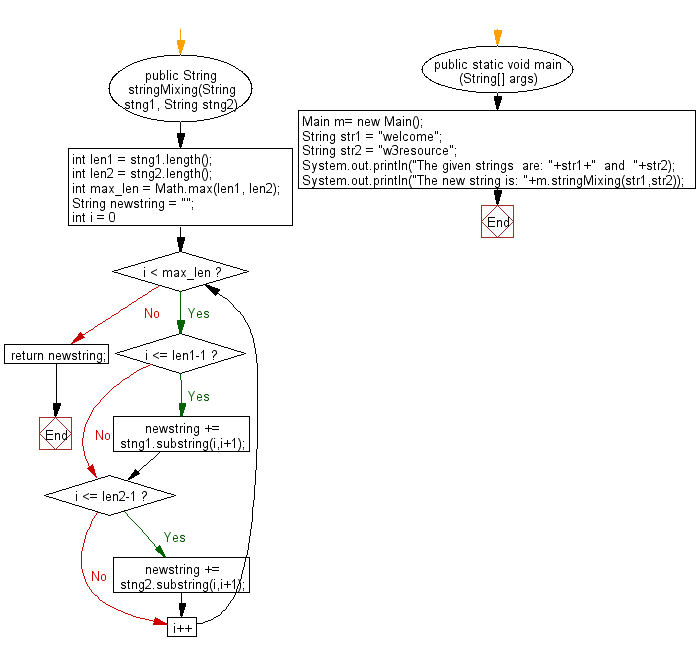﻿ Java exercises: Make a new string from two given string in such a way that, each character of two string will come respectively - w3resource# Java String Exercises: Make a new string from two given string in such a way that, each character of two string will come respectively

## Java String: Exercise-83 with Solution

Write a Java program to make a new string from two given string in such a way that, each character of two string will come respectively.

Sample Solution:

Java Code:

``````import java.util.*;
public class Main
{
public String stringMixing(String stng1, String stng2)
{
int len1 = stng1.length();
int len2 = stng2.length();
int max_len = Math.max(len1, len2);
String newstring = "";
for (int i = 0; i < max_len; i++)
{
if (i <= len1-1)
newstring += stng1.substring(i,i+1);
if (i <= len2-1)
newstring += stng2.substring(i,i+1);
}
return newstring;
}

public static void main (String[] args)
{
Main m= new Main();
String str1 =  "welcome";
String str2 =  "w3resource";
System.out.println("The given strings  are: "+str1+"  and  "+str2);
System.out.println("The new string is: "+m.stringMixing(str1,str2));
}
}
``````

Sample Output:

```The given strings  are: welcome  and  w3resource
The new string is: wwe3lrceosmoeurce
```

Pictorial Presentation:Flowchart:Java Code Editor:

Improve this sample solution and post your code through Disqus

What is the difficulty level of this exercise?

﻿

## Java: Tips of the Day

Directory Content:

Java allows you to get the names of all subdirectories and files in a folder as an array, which can then be sequentially viewed.

```import java.io.*;

public class ListContents {
public static void main(String[] args) {
File file = new File("//home//user//Documents/");
String[] files = file.list();

System.out.println("Listing contents of " + file.getPath());
for(int i=0 ; i < files.length ; i++)
{
System.out.println(files[i]);
}
}
}
```